Function Repository Resource:

# GeodesateMesh

Generate a geodesic dome from a polyhedral base

Contributed by: Wolfram Staff
 ResourceFunction["GeodesateMesh"][reg,n] generates a region representing the projection of the order-n regular tessellation of each face of the polyhedron reg onto the circumscribed sphere centered at the origin. ResourceFunction["GeodesateMesh"][reg,n,p,r] uses a circumscribed sphere centered at the point p with radius r.

## Details

ResourceFunction["GeodesateMesh"][reg] is equivalent to ResourceFunction["GeodesateMesh"][reg,2].
ResourceFunction["GeodesateMesh"] returns a BoundaryMeshRegion object if reg is a BoundaryMeshRegion or Polyhedron object.
ResourceFunction["GeodesateMesh"] returns a GraphicsComplex object if reg is also a GraphicsComplex object.
ResourceFunction["GeodesateMesh"] triangulates polygons with five or more sides before projecting them onto the circumscribed sphere.

## Examples

### Basic Examples (1)

Geodesate an icosahedron:

 In:=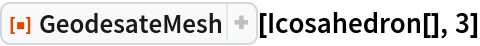Out=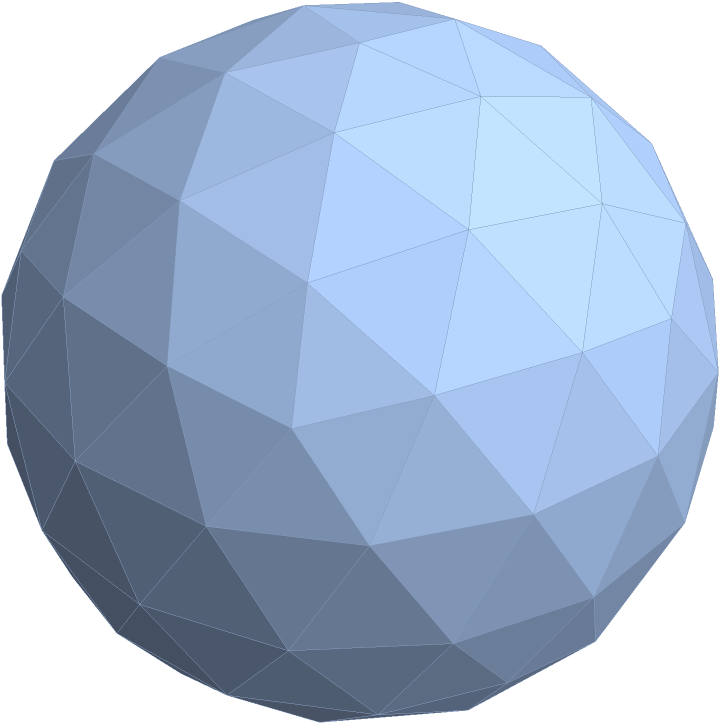### Scope (3)

Use the default geodesation order:

 In:=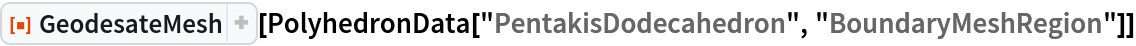Out=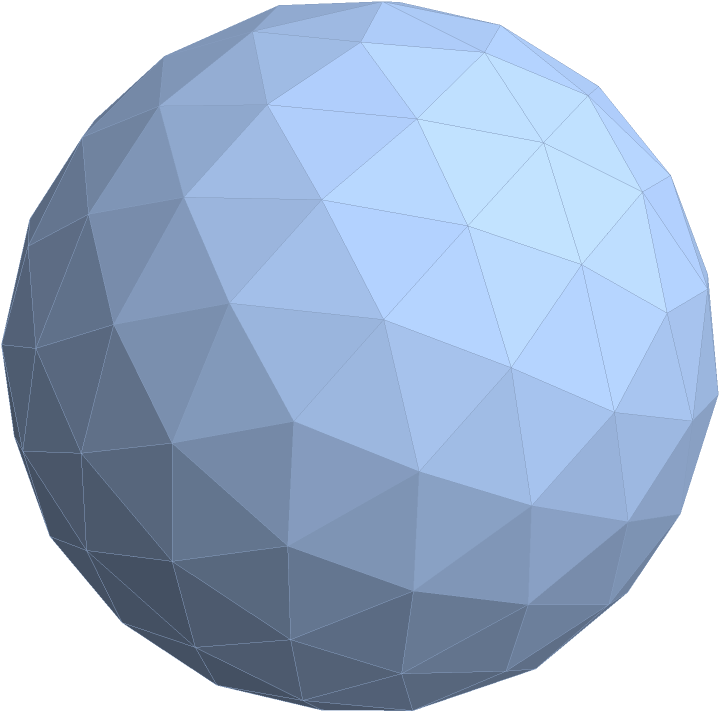Use a different center and radius for the circumscribing sphere:

 In:=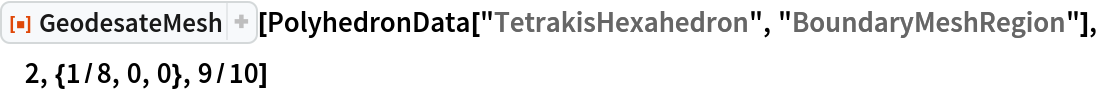Out=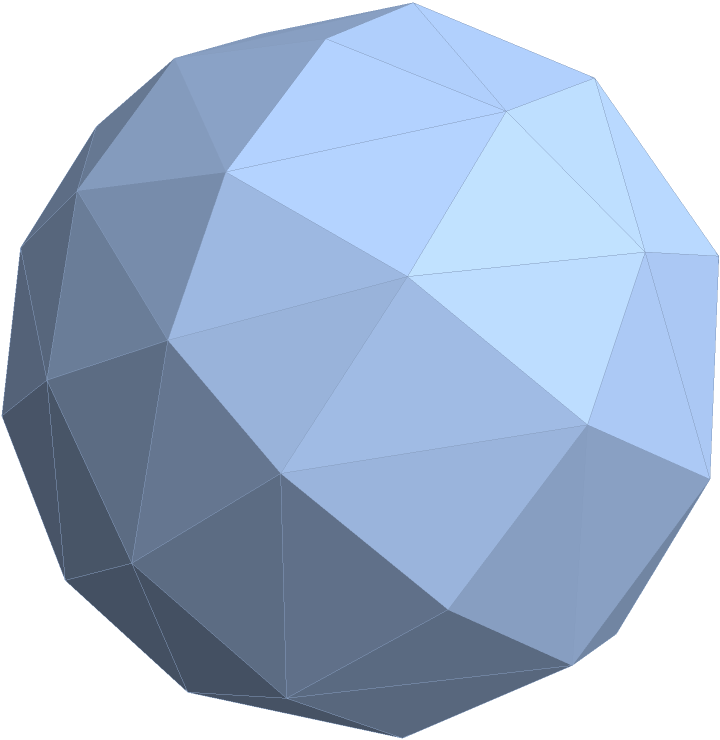Use GeodesateMesh on a GraphicsComplex object:

 In:=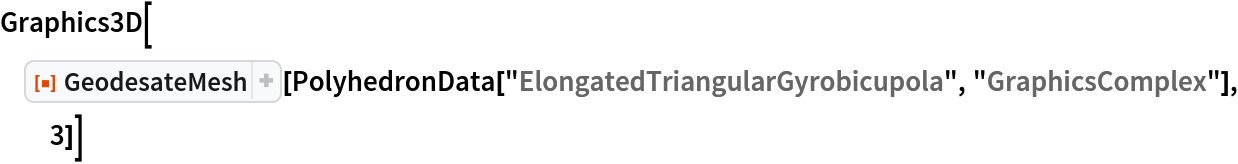Out=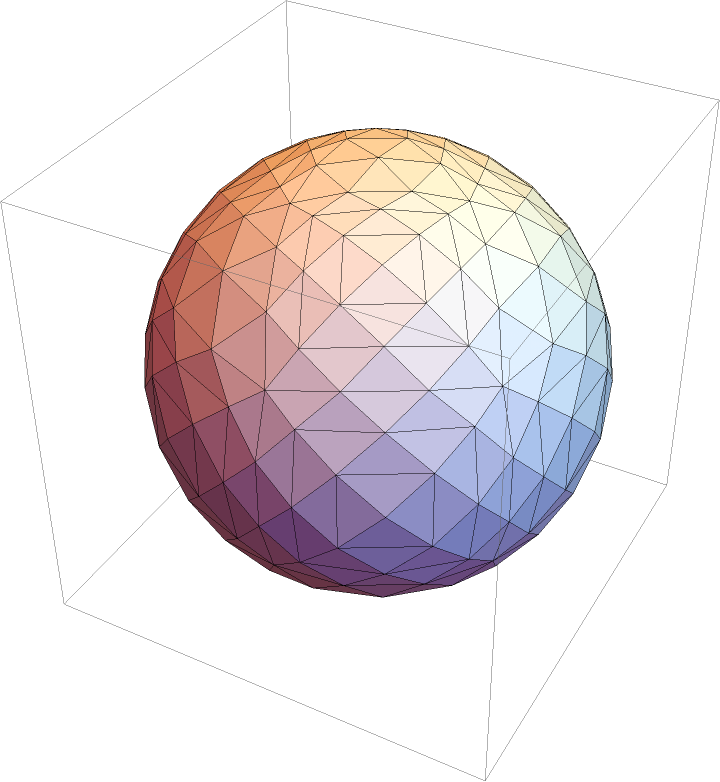### Properties and Relations (1)

Use CanonicalizePolyhedron to get a Polyhedron object that can be used in Graphics3D:

 In:=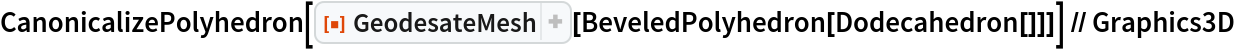Out=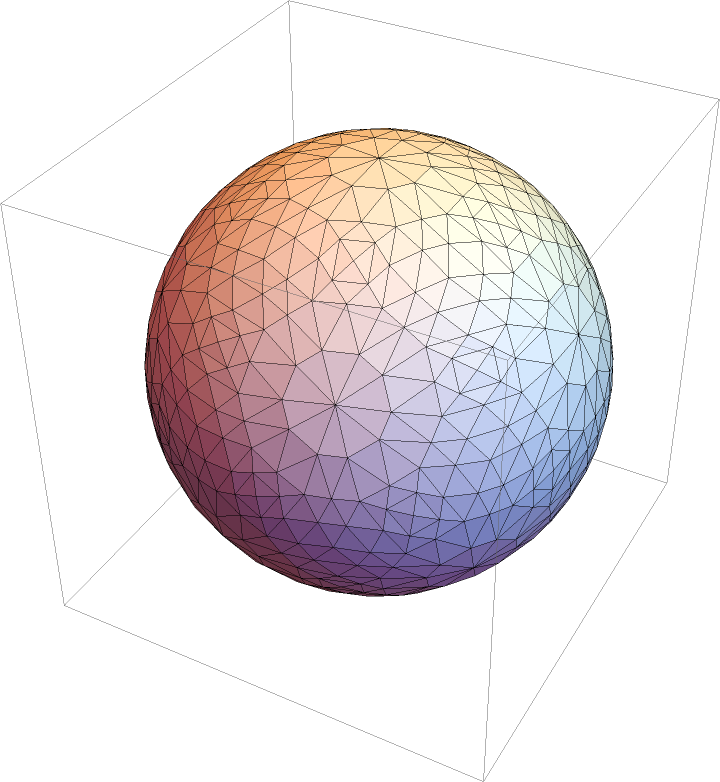### Neat Examples (1)

Visualize a Goldberg polyhedron:

 In:=Out=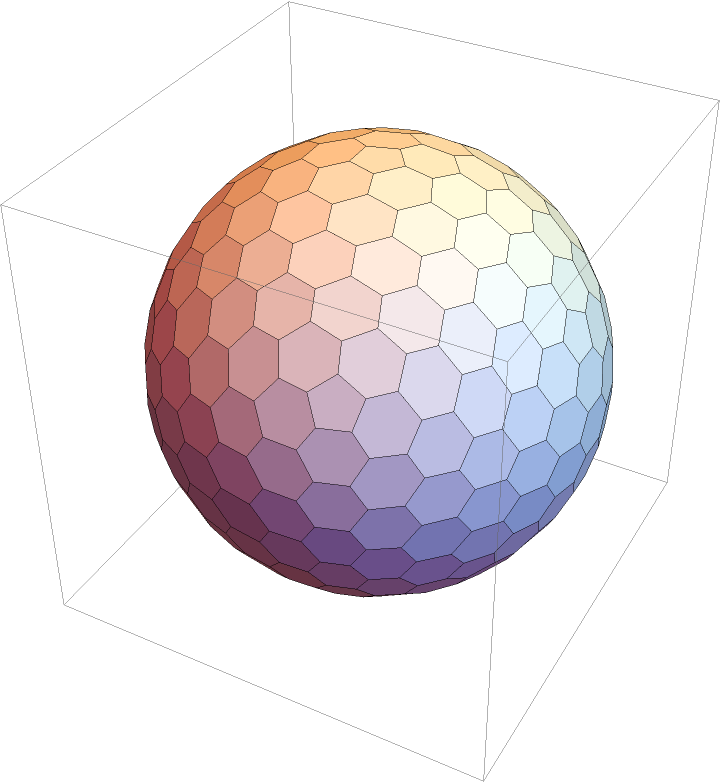## Version History

• 1.0.0 – 28 June 2021

## Author Notes

GeodesateMesh can return unexpected results if the non-triangular faces of a given polyhedron have been triangulated in advance. If using PolyhedronData, stick to the results of the "BoundaryMeshRegion" or "GraphicsComplex" properties.